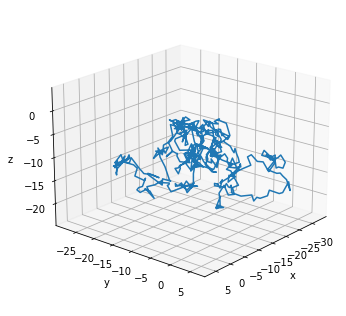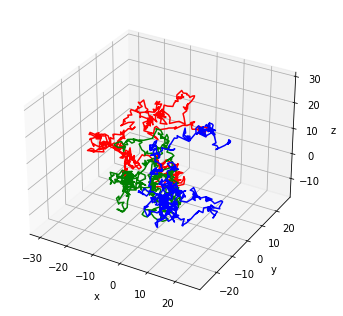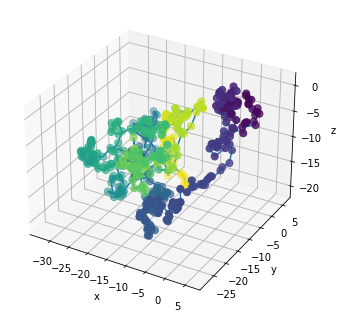# Path3D#

Title
Path3D Element
Dependencies
Matplotlib
Backends
Matplotlib
Plotly
```import numpy as np
import holoviews as hv

hv.extension('matplotlib')
```A `Path3D` element represents one more lines, connecting arbitrary points in three-dimensional space. `Path3D` supports plotting an individual line or multiple subpaths, which should be supplied as a list. Each path should be defined in a columnar format such as NumPy arrays, DataFrames or dictionaries for each column. For a full description of the path geometry data model see the Geometry Data User Guide.

As a simple example we will generate a random walk through 3D space as a single array with three columns:

```line = np.random.randn(500, 3).cumsum(axis=0)
path = hv.Path3D(line)

path.opts(azimuth=40, elevation=20)
```Like the 2D equivalent `Path` also allows drawing multiple paths and allows coloring each by a value:

```paths = [{('x', 'y', 'z'): np.random.randn(500, 3).cumsum(axis=0), 'index': i} for i in range(3)]
hv.Path3D(paths, vdims='index').opts(color='index', cmap=['red', 'green', 'blue'])
```Just like all regular 2D elements, `Path3D` types can be overlaid with other 2D and 3D elements:

```scatter = hv.Scatter3D({('x', 'y', 'z'): line, 'index': np.arange(500)}, vdims='index')

path * scatter.opts(color='index', cmap='viridis', s=50)
```For full documentation and the available style and plot options, use `hv.help(hv.Path3D).`

This web page was generated from a Jupyter notebook and not all interactivity will work on this website. Right click to download and run locally for full Python-backed interactivity.